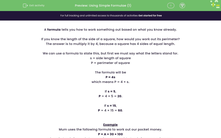Using Simple Formulae (1)

In this worksheet, students use simple formulae to answer questions. Symbols are used.Key stage:  KS 2

Curriculum topic:   Algebra

Curriculum subtopic:   Use Algebra Formulae

Difficulty level:Worksheet Overview

A formula tells you how to work something out based on what you know already.

If you know the length of the side of a square, how would you work out its perimeter?

The answer is to multiply it by 4, because a square has 4 sides of equal length.

We can use a formula to state this, but first we must say what the letters stand for.

s = side length of square

P = perimeter of square

The formula will be

P = 4s

which means P = 4 × s.

If s = 5,

P = 4 × 5 = 20.

If s = 15,

P = 4 × 15 = 60.

Example

Mum uses the following formula to work out our pocket money.

P = A × 30 + 100

where P stands for pocket money in pence and A stands for our age in years.

Bill is 5 years old. How much does he get?

Amy is 15 years old. How much does she get?

Bill

A = 5

P = 5 × 30 + 100 = 150 + 100 = 250 pence

Amy

A = 15

P = 15 × 30 + 100 = 450 + 100 = 550 pence

What is EdPlace?

We're your National Curriculum aligned online education content provider helping each child succeed in English, maths and science from year 1 to GCSE. With an EdPlace account you’ll be able to track and measure progress, helping each child achieve their best. We build confidence and attainment by personalising each child’s learning at a level that suits them.

Get started